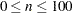The STDIZE Procedure
 Output Data Sets

OUT= Data Set

The output data set is a copy of the DATA= data set except that the analyzed variables have been standardized. Analyzed variables are those listed in the VAR statement or, if there is no VAR statement, all numeric variables not listed in any other statement.

OUTSTAT= Data Set

The new data set contains the following variables:

• the BY variables, if any

• _TYPE_, a character variable

• the analyzed variables

Each observation in the new data set contains a type of statistic as indicated by the _TYPE_ variable. The values of the _TYPE_ variable are as follows:

LOCATION

location measure of each variable

SCALE

scale measure of each variable

constant specified in the ADD= option. This value is the same for each variable.

MULT

constant specified in the MULT= option. This value is the same for each variable.

N

total number of nonmissing positive frequencies of each variable

NORM

norm measure of each variable. This observation is produced only when you specify the NORM option with METHOD=AGK, METHOD=IQR, METHOD=MAD, or METHOD=SPACING or when you specify the SNORM option with METHOD=SPACING.

NObsUsed

number of physical records used in the analysis

NObsMiss

number of physical records containing missing values

Pn

percentiles of each variable, as specified by the PCTLPTS= option. The argument n is any real number such thatsum of the frequency variable (or the sum of NObsUsed ones when there is no frequency variable) for all observations read

SumFreqsUsed

sum of the frequency variable (or the sum of NObsUsed ones when there is no frequency variable) for all observations used in the analysis